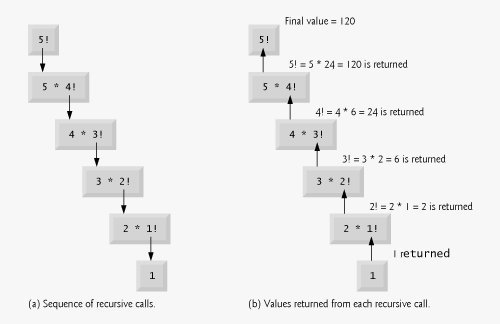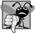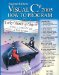# Recursion

The applications we have discussed thus far are generally structured as methods that call one another in a disciplined, hierarchical manner. For some problems, however, it is useful to have a method call itself. A recursive method is a method that calls itself, either directly or indirectly through another method.

We consider recursion conceptually first. Then we examine an application containing a recursive method. Recursive problem-solving approaches have a number of elements in common. When a recursive method is called to solve a problem, the method actually is capable of solving only the simplest case(s), or base case(s). If the method is called with a base case, the method returns a result. If the method is called with a more complex problem, the method divides the problem into two conceptual pieces: a piece that the method knows how to do and a piece that the method does not know how to do. To make recursion feasible, the latter piece must resemble the original problem, but be a slightly simpler or slightly smaller version of it. Because this new problem looks like the original problem, the method calls a fresh copy of itself to work on the smaller problem; this is referred to as a recursive call and is also called the recursion step. The recursion step normally includes a return statement, because its result will be combined with the portion of the problem the method knew how to solve to form a result that will be passed back to the original caller.

The recursion step executes while the original call to the method is still active (i.e., while it has not finished executing). The recursion step can result in many more recursive calls, as the method divides each new subproblem into two conceptual pieces. For the recursion to terminate eventually, each time the method calls itself with a slightly simpler version of the original problem, the sequence of smaller and smaller problems must converge on the base case. At that point, the method recognizes the base case and returns a result to the previous copy of the method. A sequence of returns ensues until the original method call returns the result to the caller. This process sounds complex compared with the conventional problem solving we have performed to this point.

Recursive Factorial Calculations

As an example of recursion concepts at work, let us write a recursive application to perform a popular mathematical calculation. Consider the factorial of a nonnegative integer n, written n! (and pronounced "n factorial"), which is the product

n · (n 1) · (n 2) · ... · 1

1! is equal to 1 and 0! is defined to be 1. For example, 5! is the product 5 · 4 · 3 · 2 · 1, which is equal to 120.

The factorial of an integer, number, greater than or equal to 0 can be calculated iteratively (nonrecursively) using the for statement as follows:

```factorial = 1;

for ( int counter = number; counter >= 1; counter-- )
factorial *= counter;
```

A recursive declaration of the factorial method is arrived at by observing the following relationship:

n! = n · (n 1)!

For example, 5! is clearly equal to 5 · 4!, as is shown by the following equations:

5! = 5 · 4 · 3 · 2 · 1

5! = 5 · (4 · 3 · 2 · 1)

5! = 5 · (4!)

The evaluation of 5! would proceed as shown in Fig. 7.16. Figure 7.16(a) shows how the succession of recursive calls proceeds until 1! is evaluated to be 1, which terminates the recursion. Figure 7.16(b) shows the values returned from each recursive call to its caller until the value is calculated and returned.

Figure 7.16. Recursive evaluation of 5!.Figure 7.17 uses recursion to calculate and print the factorials of the integers from 0 to 10. The recursive method Factorial (lines 1624) first tests to determine whether a terminating condition (line 19) is TRue. If number is less than or equal to 1 (the base case), Factorial returns 1, no further recursion is necessary and the method returns. If number is greater than 1, line 23 expresses the problem as the product of number and a recursive call to Factorial evaluating the factorial of number -1, which is a slightly simpler problem than the original calculation, Factorial( number ).

Figure 7.17. Recursive Factorial method.

(This item is displayed on page 306 in the print version)

``` 1 // Fig. 7.17: FactorialTest.cs
2 // Recursive Factorial method.
3 using System;
4
5 public class FactorialTest
6 {
7 public static void Main( string[] args )
8 {
9 // calculate the factorials of 0 through 10
10 for ( long counter = 0; counter <= 10; counter++ )
11 Console.WriteLine( "{0}! = {1}",
12 counter, Factorial( counter ) );
13 } // end method Main
14
15 // recursive declaration of method Factorial
16 public static long Factorial( long number )
17 {
18 // base case
19 if ( number <= 1 )
20 return 1;
21 // recursion step
22 else
23 return number * Factorial( number - 1 );
24 } // end method Factorial
25 } // end class FactorialTest
```

 ```0! = 1 1! = 1 2! = 2 3! = 6 4! = 24 5! = 120 6! = 720 7! = 5040 8! = 40320 9! = 362880 10! = 3628800 ```

Method Factorial (lines 1624) receives a parameter of type long and returns a result of type long. As can be seen in Fig. 7.17, factorial values become large quickly. We chose type long (which can represent relatively large integers) so that the application could calculate factorials greater than 20!. Unfortunately, the Factorial method produces large values so quickly that factorial values soon exceed even the maximum value that can be stored in a long variable. Due to the restrictions on the integral types, variables of type float, double, and decimal might ultimately be needed to calculate factorials of larger numbers. This situation points to a weakness in many programming languagesthe languages are not easily extended to handle the unique requirements of various applications. As you know, C# is an extensible language that allows you to create a type that supports arbitrarily large integers if you wish. You could create a HugeInteger class, for example, that could enable an application to calculate the factorials of arbitrarily large numbers.## Common Programming Error 7 11

Either omitting the base case or writing the recursion step incorrectly so that it does not converge on the base case will cause infinite recursion, eventually exhausting memory. This error is analogous to the problem of an infinite loop in an iterative (nonrecursive) solution.Visual C# 2005 How to Program (2nd Edition)
ISBN: 0131525239
EAN: 2147483647
Year: 2004
Pages: 600